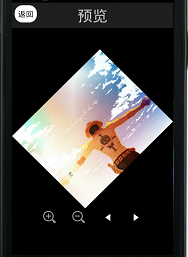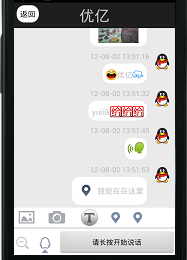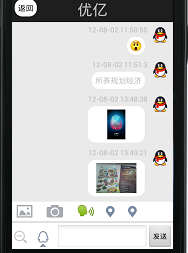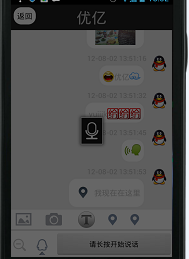继人员列表，聊天的实现，包括图片，语音，位置，表情

年薪达不到25W大数据工程师、拿不到Offer全额退款->>>eoeandroid wiki

http://wiki.eoeandroid.com/%E9%A6%96%E9%A1%B5

eoeandroid 招聘

http://zhaopin.eoe.cn/?from=forum

Android资源汇总贴，给力！private String table = "CREATE TABLE IF NOT EXISTS message(" + "_id integer PRIMARY KEY," + "TtmType integer ," + "TtmTuID integer ," + "TtmToUserId integer ," + "TtmContent text ," + "TtmTime varchar(200) ," + "isRead integer ," + "isReplyLocation integer )";

public List<DetailEntity> getdatas( int TtmTuID, int TtmToUserId) { List<DetailEntity> de = new ArrayList<DetailEntity> (); SQLiteDatabase database = dbmanger.getWritableDatabase(); Cursor cursor = database.rawQuery( "select * from message where (TtmTuID = ? and TtmToUserId = ?) or (TtmToUserId = ? and TtmTuID = ?) order by _id" , new String[] { String.valueOf(TtmTuID), String.valueOf(TtmToUserId), String.valueOf(TtmTuID), String.valueOf(TtmToUserId)}); while (cursor.moveToNext()) { de.add( new DetailEntity(cursor.getInt(1), cursor.getInt(2 ), cursor .getInt(3), cursor.getString(4), cursor.getString(5),cursor.getInt(6),cursor.getInt(7 ))); } cursor.close(); database.close(); return de; }

messageList = MessageSQLService.getInstance( this ).getdatas( Integer.valueOf(UserInfomation.getUserID(TalkMessageAct. this )), Integer.valueOf(TFuid)); for ( int i = 0; i < messageList.size(); i++ ) { switch (messageList.get(i).getTtmType()) { case 1 : int id = Integer.valueOf(messageList.get(i).getTtmTuID()); if (id == Integer.valueOf(TFuid)) { } else if (id == Integer.valueOf(UserInfomation .getUserID(TalkMessageAct. this ))) { } break ; case 2 : int ids = Integer.valueOf(messageList.get(i).getTtmTuID()); if (ids == Integer.valueOf(TFuid)) { } else if (ids == Integer.valueOf(UserInfomation .getUserID(TalkMessageAct. this ))) { } break ; case 3 : int idsx = Integer.valueOf(messageList.get(i).getTtmTuID()); if (idsx == Integer.valueOf(TFuid)) { } else if (idsx == Integer.valueOf(UserInfomation .getUserID(TalkMessageAct. this ))) { } break ; case 5 : break ; case 6 : int idsxx = Integer.valueOf(messageList.get(i).getTtmTuID()); if (idsxx == Integer.valueOf(TFuid)) { } else if (idsxx == Integer.valueOf(UserInfomation .getUserID(TalkMessageAct. this ))) { } break ; }}

socket = new Socket(Constants.SOCKET_IP, Constants.SOCKET_PORT); if (socket.isConnected()) { out = new PrintWriter( new BufferedWriter( new OutputStreamWriter(socket.getOutputStream())), true ); out.write("Lgn|"+ UserInfomation.getUserID(TalkMessageServer. this)+"|"+ UserInfomation.getUserPW(TalkMessageServer. this )); out.flush(); socket.shutdownOutput(); } reader = new BufferedReader( new InputStreamReader( socket.getInputStream())); while ((line = reader.readLine()) != null ) { if (line != null ) { // 截取字符，读取信息的操作 }

Test.rar